# Seaborn BoxPlot

Manav Narula Oct 12, 2021 May 22, 2021

Different statistical graphs and plots can be created using the seaborn module in Python. We can create a box plot using the `seaborn.boxplot()` function.

Analysts and statisticians frequently use a box plot to represent data across different levels or categories. The box plot figure can convey many statistical values. The following figure explains this.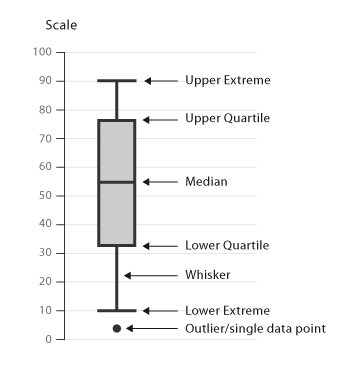The `boxplot()` function works efficiently in plotting categorical values of a dataset and can also work with single lists or array vectors. It is very similar to the violin plot.

In the following example, we plot a box plot for a single distribution to get some idea of the final figure.

``````import random
import numpy as np
import seaborn as sns

n = random.sample(range(0,50),30)
arr = np.array(n)
sns.boxplot(n)
``````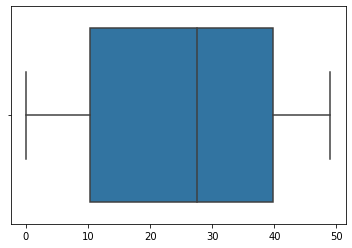We can also plot a scatter plot over the box plot. It will give you a better understanding of the plotted distribution and the final figure.

We will use the `seaborn.stripplot()` function to plot the scatter graph. For example,

``````import random
import numpy as np
import seaborn as sns

n = random.sample(range(0,50),30)
arr = np.array(n)
sns.boxplot(n)
sns.stripplot(n, color = 'red')
``````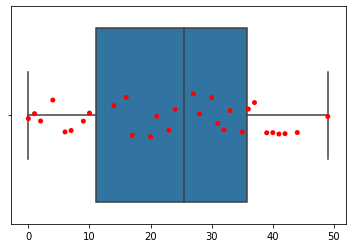The box plot can be efficiently utilized to plot the categorical variables and make their comparison simple. In the following code, we will plot the box plot for multiple categories.

``````import pandas as pd
import matplotlib.pyplot as plt
import seaborn as sns

df = pd.DataFrame({"Quantity": [5,6,7,8,5,6,7,8,5,6,7,8,5,6,7,8],
"Price": [9,10,15,16,13,14,15,18,11,12,14,15,16,17,18,19],
"Day" : [1,1,1,1,1,1,1,1,2,2,2,2,2,2,2,2],
"Product": ['A','A','A','A','B','B','B','B',
'A','A','A','A','B','B','B','B']})

sns.boxplot(data = df, y = "Price", x = "Quantity")
``````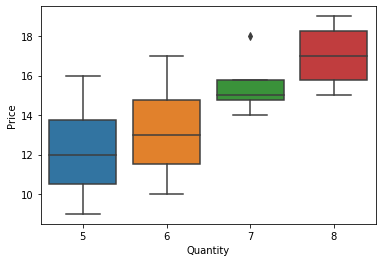Notice how in the above dataset, we can easily compare the price across different quantities. We can also add the `hue` parameter and set its value as the `Product` variable. This way, we will be able to plot different boxes for different products.

We can use the `matplotlib.pyplot.ylim()` and `matplotlib.pyplot.xlim()` to set the limits on x and the y axis of the plot.

We can use many more parameters to tweak the final plot. The `linewidth` parameter can be used to increase the thickness of the borders of the box plot. We can customize the colors for different categories using the `palette` parameter. The orientation can be changed using the `orient` argument. There are many more such arguments that can be used.

See the code below to understand the application of some of these parameters.

``````import pandas as pd
import matplotlib.pyplot as plt
import seaborn as sns

df = pd.DataFrame({"Quantity": [5,6,7,8,5,6,7,8,5,6,7,8,5,6,7,8],
"Price": [9,10,15,16,13,14,15,18,11,12,14,15,16,17,18,19],
"Day" : [1,1,1,1,1,1,1,1,2,2,2,2,2,2,2,2],
"Product": ['A','A','A','A','B','B','B','B',
'A','A','A','A','B','B','B','B']})

sns.boxplot(data = df, y = "Price", x = "Quantity", hue = 'Product', linewidth = 2.5 , palette = 'Set2')
``````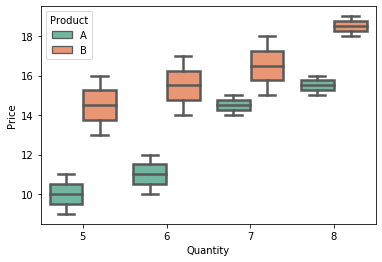We can also use the `catplot()` function to create a box plot. The `catplot()` function works very well for plotting categorical values. For creating a box plot using this function, we need to specify the value of the `kind` parameter in the `catplot()` function as `box`.

Author: Manav Narula

Manav is a IT Professional who has a lot of experience as a core developer in many live projects. He is an avid learner who enjoys learning new things and sharing his findings whenever possible.